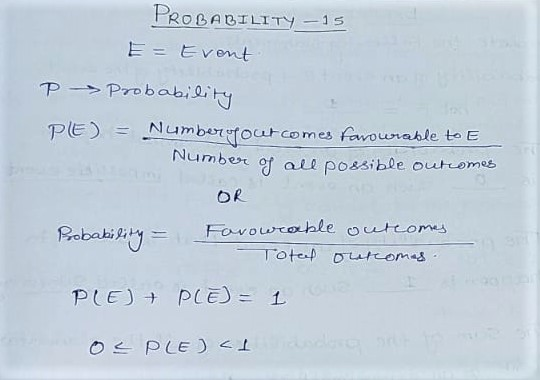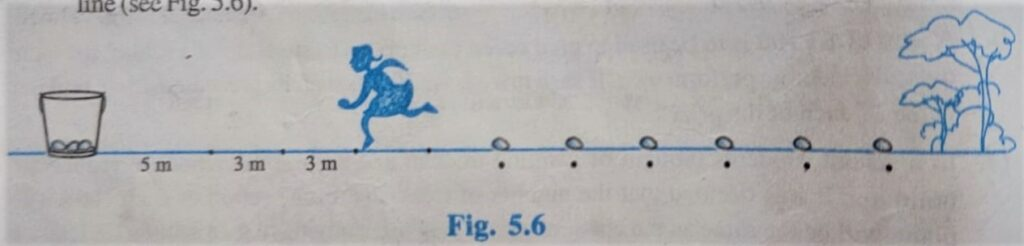# Mathematics## NCERT Solution Exercise 15.1 Probability Class 10

Q1. Complete the Following Statements: (i) Probability of an event E + Probability of the event ‘not E’ = 1 (ii) The Probability of an event that can not happen is  0 such an event is called an impossible event. (iii) The probability of an event that is certain to happen is 1 such an event is …

## NCERT Solution Exercise – 6.2 Class 10

Q.1 In fig.6.17,(i)and(ii),DE || BC. find EC in(i)and AD in (ii) (i)  Solution: According to figure (i) in ΔABC DE || BC { given } By B.P.T. [ In a triangle if a line is parallel to one side divides remaining to sides in equal ratio ] AD / BD = AE / EC 1.5/3 …

## NCERT Solution Exercise 5.1 Arithmetic progressions Class-10

Arithmetic progressions: Basic concepts Sequence: Some numbers arranged in a definite order, according to a definite rule, are said to form a sequence. ex   (I) 1, 2, 3,……… (II) 100, 70, 40, 10 ……… (III) -1·0, -1·5, -2·0, -2·5, ……. Arithmatic progressions (AP): An arithmetic progression is a list of numbers in which each term …## NCERT Solution Exercise – 4.3 Class – 10 Maths

Quadratic formula / Shreedharacharya sutra:  x=-b±√b²-4ac/2a  For Finding Nature of roots: First we find Discriminant (D) : b²- 4ac There are three different cases Case I : b²-4ac<0 Nature of Roots :  Imaginary Roots/ No Real roots Case II : b² – 4ac = 0 Nature of Roots : Real and two different roots Case III …

## NCERT Solutions Exercise 4.1 Class 10 Maths

Q.1. Check whether the following are quadratic equations ÷ (i) (x + 1)2 = 2 (x – 3) Solution: (x + 1)2 = 2 (x – 3) [By Applying ( a + b )² = a²+2ab+b2] ⇒ x²+2x+1= 2x – 6 ⇒ x²+2x+1 – 2x + 6 = 0 ⇒ x² + 7  = 0 …## NCERT Solution Exercise 5.3 Class 10 Maths

Important formula for this Exercise: Sum of n terms of AP= Sn  (i) Sn = n/2[2a+(n-1)d] (ii) Sn = n/2[a + an ] (iii) Sn = n/2[a + l ] (iv) Sum of first n positive integers = Sn = n(n+1)/2 Q.1 Find the sum of the Following APs; (i) 2, 7,12,…………to 10 terms a = first term = 2 …